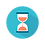From: Bayesian Models for Astrophysical Data, Cambridge Univ. Press

(c) 2017,  Joseph M. Hilbe, Rafael S. de Souza and Emille E. O. Ishida

you are kindly asked to include the complete citation if you used this material in a publication

Code 5.31 Simulated beta–binomial data in R

========================================================

# Required packages
require(R2jags)
require(boot)
require(VGAM)

# Simulation
set.seed(33559)
nobs = 2500

m = 1 + rpois(nobs,5)
x1 = runif(nobs)

beta1 <- -2
beta2 <- -1.5

eta <- beta1 + beta2 * x1
sigma <- 20

p <- inv.logit(eta)

shape1 = sigma*p
shape2 = sigma*(1 - p)

y <- rbetabinom.ab(n=nobs, size=m, shape1=shape1, shape2=shape2)

bindata = data.frame(y=y,m=m,x1)

========================================================

Code 5.32  Beta–binomial synthetic model in R using JAGS

========================================================

X <- model.matrix(~ x1, data = bindata)
K <- ncol(X)

model.data <- list(Y = bindata\$y,
N = nrow(bindata),
X = X,
K = K,
m = m )

sink("GLOGIT.txt")

cat("
model{
# Diffuse normal priors betas
for (i in 1:K) { beta[i] ~ dnorm(0, 0.0001)}

# Prior for theta
sigma ~ dgamma(0.01,0.01)

for (i in 1:N){
Y[i] ~ dbin(p[i],m[i])
p[i] ~ dbeta(shape1[i],shape2[i])

shape1[i] <- sigma*pi[i]
shape2[i] <- sigma*(1-pi[i])
logit(pi[i]) <- eta[i]
eta[i] <- inprod(beta[],X[i,])
}
}
"
,fill = TRUE)

sink()

inits <- function () {list(beta = rnorm(K, 0, 0.1)) }

params <- c("beta", "sigma")

BBIN <- jags(data = model.data,
inits = inits,
parameters = params,
model.file = "GLOGIT.txt",
n.thin = 1,
n.chains = 3,
n.burnin = 3000,
n.iter = 5000)

print(BBIN, intervals=c(0.025, 0.975), digits=3)

========================================================

GET SOURCEOutput on screen:

Inference for Bugs model at "GLOGIT.txt", fit using

jags, 3 chains, each with 5000 iterations (first 3000 discarded)

n.sims = 6000 iterations saved

mu.vect     sd.vect             2.5%            97.5%      Rhat     n.eff

beta               -1.948       0.063           -2.075           -1.827     1.008     1500

beta               -1.595       0.129           -1.833           -1.322      1.004      950

sigma                20.539       3.528           14.671          28.577      1.013      380

deviance       3337.284      74.220      3188.404      3477.857      1.011       380

For each parameter, n.eff is a crude measure of effective sample size,

and Rhat is the potential scale reduction factor (at convergence, Rhat=1).

DIC info (using the rule, pD = var(deviance)/2)

pD = 2741.5 and DIC = 6078.8

DIC is an estimate of expected predictive error (lower deviance is better).